# Nichols Chart: What is it?

## What is a Nichols Chart?

A Nichols Chart (also known as a Nichols Plot) is a plot used in signal processing and control system design to determine the stability and closed-loop frequency response of a feedback system. The Nichols chart is named after its founder, Nathaniel B. Nichols.

## How Does A Nichols Chart Work?

Constant magnitude loci that are M-circles and constant phase angle loci that are N-circles are the fundamental components in designing the Nichols chart.

The constant M and constant N circles in the G (jω) plane can be used to analyze and design control systems.

However, the constant M and constant N circles in the gain phase plane are prepared for system design and analysis as these plots supply information with fewer manipulations.

The gain phase plane is the graph having gain in decibels along the ordinate (vertical axis) and phase angle along the abscissa (horizontal axis).

The M and N circles of G (jω) in the gain phase plane are transformed into M and N contours in rectangular coordinates.

A point on the constant M loci in G (jω) plane is transferred to the gain phase plane by drawing the vector directed from the origin of the G (jω) plane to a particular point on the M circle and then measuring the length in dB and angle in degree.

The critical point in G (jω), plane corresponds to the point of zero decibels and -180o in the gain phase plane. The plot of M and N circles in the gain phase plane is known as the Nichols chart (or Nichols plot).

Compensators can be designed using a Nichols plot.

The Nichols plot technique is also used in the design of a DC motor. This is used in signal processing and control system design.

The related Nyquist plot in the complex plane shows how the phase of the transfer function and frequency variation of magnitude are related. We can find out the gain and phase for a given frequency.

The angle of the positive real axis determines the phase and the distance from the origin of the complex plane determines the gain. There are some advantages of Nichols’ plot in control system engineering.

They are:

• Gain and phase margins can be determined easily and also graphically.
• Closed loop frequency response is obtained from open loop frequency response.
• The gain of the system can be adjusted to suitable values.
• Nichols chart provides frequency domain specifications.

There are also some drawbacks to the Nichols plot. Using a Nichols plot is difficult for small changes in gain.

Constant M and N circles in the Nichols chart are deformed into squashed circles.

The complete Nichols chart extends for the phase angle of G (jω) from 0 to -360o. The region of ∠G(jω) is used for the analysis of systems between -90o to -270o. These curves repeat after every 180o interval.

If the open loop T.F of unity feedback system G(s) is expressed as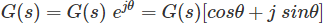Closed loop T.F is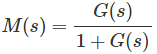Substituting s = jω in the above eq. frequency functions are,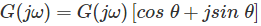and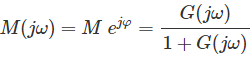Eliminating G(jω) from the above two eq.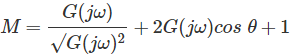and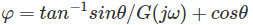Want To Learn Faster? 🎓
Get electrical articles delivered to your inbox every week.
No credit card required—it’s 100% free.